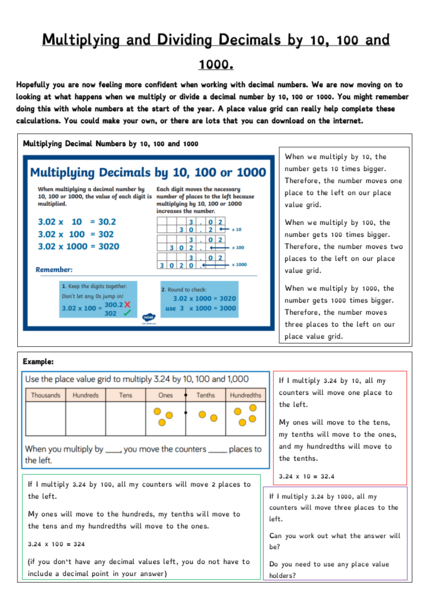# Multiplying and Dividing DecimalsContributed by:In this pdf, we will be Multiplying and Dividing Decimals by 10, 100 and 1000.
1. Multiplying and Dividing Decimals by 10, 100 and
1000.
Hopefully you are now feeling more confident when working with decimal numbers. We are now moving on to
looking at what happens when we multiply or divide a decimal number by 10, 100 or 1000. You might remember
doing this with whole numbers at the start of the year. A place value grid can really help complete these
calculations. You could make your own, or there are lots that you can download on the internet.
Multiplying Decimal Numbers by 10, 100 and 1000
When we multiply by 10, the
number gets 10 times bigger.
Therefore, the number moves one
place to the left on our place
value grid.
When we multiply by 100, the
number gets 100 times bigger.
Therefore, the number moves two
places to the left on our place
value grid.
When we multiply by 1000, the
number gets 1000 times bigger.
Therefore, the number moves
three places to the left on our
place value grid.
Example:
If I multiply 3.24 by 10, all my
counters will move one place to
the left.
My ones will move to the tens,
my tenths will move to the ones,
and my hundredths will move to
the tenths.
3.24 x 10 = 32.4
If I multiply 3.24 by 100, all my counters will move 2 places to
the left. If I multiply 3.24 by 1000, all my
counters will move three places to the
My ones will move to the hundreds, my tenths will move to
left.
the tens and my hundredths will move to the ones.
Can you work out what the answer will
3.24 x 100 = 324
be?
(if you don’t have any decimal values left, you do not have to Do you need to use any place value
2. Dividing Decimal Numbers by 10, 100 and 1000
When we divide by 10, the
number gets 10 times smaller.
Therefore, the number moves one
place to the right on our place
value grid.
When we divide by 100, the
number gets 100 times smaller.
Therefore, the number moves two
places to the right on our place
value grid.
When we multiply by 1000, the
number gets 1000 times smaller.
Therefore, the number moves
three places to the right on our
place value grid.
If I divide 14.4 by 10, all my counters will
move one place to the right.
My tenths will move to hundredths, my
ones will move to tenths and my tens will
move to the ones.
14.4 ÷ 10 = 1.44
If I divide 14.4 by 100, all my counters will move two
If I divide 14.4 by 1000, all my counters
places to the right.
will move three places to the right.
My tenths will become thousandths, my ones will become
Can you work out what the answer will
hundredths and my tens will become tenths.
be?
14.4 ÷ 100 + 0.144
Where will your place holders need to
Now I no longer have any ones I use 0 as a place holder. go?
Useful videos
This video focuses on whole numbers, rather than decimals. However, it does remind you the rules we use when
we multiply or divide a number by 10 and 100. If you are not confident, I would suggest watching this before
moving onto decimals: https://www.bbc.co.uk/bitesize/topics/z36tyrd/articles/z2fkwxs
In this video, they talk you through multiplying and dividing decimals by 10, 100 and 1000. It might be helpful to
have a pencil and paper or place value grid to hand when you watch it: https://www.youtube.com/watch?v=6fLNcGSa_L4
This video demonstrates how you can use a place value grid to help you:
3. Multiplying and Dividing Decimals - Challenges
We know that lots of you like to take on a challenge during our maths lessons. These challenges are not
compulsory, but are there for anyone who wishes to give them a go. Good luck!
All of these challenges were taken from the
White Rose Maths Hub. They have also
produced a ‘Home Learning’ section to their